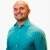# Simplifying a rational expression by factoring out the numeratorThis lesson will look at an example involving factoring. The given information will assist in learning how to simplify a rational expression by factoring out the numerator. This lesson will give a step by step explanation... This lesson will look at an example involving factoring. The given information will assist in learning how to simplify a rational expression by factoring out the numerator. This lesson will give a step by step explanation to better explain the concept.
More... Collapse